Posted by: Dan Oved, freelance creative technologist at Google Creative Lab, graduate student at ITP, NYU.
Editing and illustrations:
Irene Alvarado, creative technologist and Alexis Gallo, freelance graphic designer, at Google Creative Lab

In collaboration with Google Creative Lab, I’m excited to announce the release of a TensorFlow.js version of PoseNet¹,² a machine learning model which allows for real-time human pose estimation in the browser. Try a live demo here.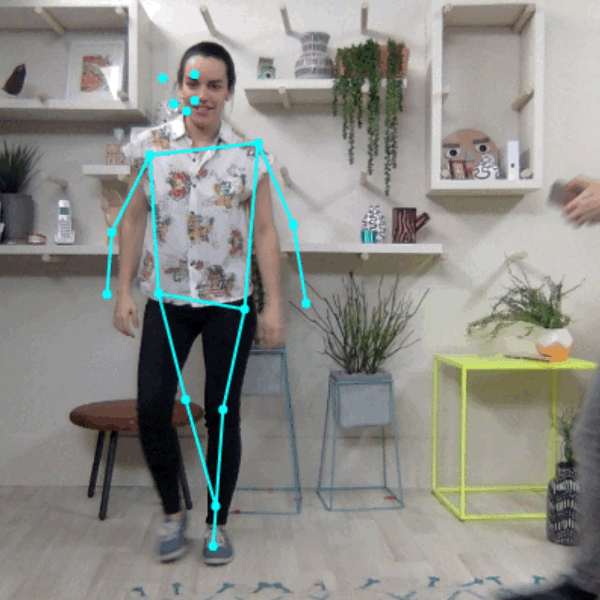PoseNet can detect human figures in images and videos using either a single-pose or multi-pose algorithm — all from within the browser.

So what is pose estimation anyway? Pose estimation refers to computer vision techniques that detect human figures in images and video, so that one could determine, for example, where someone’s elbow shows up in an image. To be clear, this technology is not recognizing who is in an image — there is no personal identifiable information associated to pose detection. The algorithm is simply estimating where key body joints are.

Ok, and why is this exciting to begin with? Pose estimation has many uses, from interactive installations that react to the body to augmented reality, animation, fitness uses, and more. We hope the accessibility of this model inspires more developers and makers to experiment and apply pose detection to their own unique projects. While many alternate pose detection systems have been open-sourced, all require specialized hardware and/or cameras, as well as quite a bit of system setup. With PoseNet running on TensorFlow.js anyone with a decent webcam-equipped desktop or phone can experience this technology right from within a web browser. And since we’ve open sourced the model, Javascript developers can tinker and use this technology with just a few lines of code. What’s more, this can actually help preserve user privacy. Since PoseNet on TensorFlow.js runs in the browser, no pose data ever leaves a user’s computer.

Before we dig into the details of how to use this model, a shoutout to all the folks who made this project possible: George Papandreou and Tyler Zhu, Google researchers behind the papers Towards Accurate Multi-person Pose Estimation in the Wild and PersonLab: Person Pose Estimation and Instance Segmentation with a Bottom-Up, Part-Based, Geometric Embedding Model, and Nikhil Thorat and Daniel Smilkov, engineers on the Google Brain team behind the TensorFlow.js library.

Getting Started with PoseNet

PoseNet can be used to estimate either a single pose or multiple poses, meaning there is a version of the algorithm that can detect only one person in an image/video and one version that can detect multiple persons in an image/video. Why are there two versions? The single person pose detector is faster and simpler but requires only one subject present in the image (more on that later). We cover the single-pose one first because it’s easier to follow.

At a high level pose estimation happens in two phases:

1. An input RGB image is fed through a convolutional neural network.
2. Either a single-pose or multi-pose decoding algorithm is used to decode poses, pose confidence scores, keypoint positions, and keypoint confidence scores from the model outputs.

But wait what do all these keywords mean? Let’s review the most important ones:

• Pose — at the highest level, PoseNet will return a pose object that contains a list of keypoints and an instance-level confidence score for each detected person.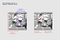PoseNet returns confidence values for each person detected as well as each pose keypoint detected. Image Credit: “Microsoft Coco: Common Objects in Context Dataset”, https://cocodataset.org.
• Pose confidence score — this determines the overall confidence in the estimation of a pose. It ranges between 0.0 and 1.0. It can be used to hide poses that are not deemed strong enough.
• Keypoint — a part of a person’s pose that is estimated, such as the nose, right ear, left knee, right foot, etc. It contains both a position and a keypoint confidence score. PoseNet currently detects 17 keypoints illustrated in the following diagram: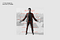Seventeen pose keypoints detected by PoseNet.
• Keypoint Confidence Score — this determines the confidence that an estimated keypoint position is accurate. It ranges between 0.0 and 1.0. It can be used to hide keypoints that are not deemed strong enough.
• Keypoint Position — 2D x and y coordinates in the original input image where a keypoint has been detected.

Part 1: Importing the TensorFlow.js and PoseNet Libraries

A lot of work went into abstracting away the complexities of the model and encapsulating functionality into easy-to-use methods. Let’s go over the basics of how to setup a PoseNet project.

The library can be installed with npm:

npm install @tensorflow-models/posenet

and imported using es6 modules:

import * as posenet from '@tensorflow-models/posenet';const net = await posenet.load();

or via a bundle in the page:

<html>
<body>
<!-- Load TensorFlow.js -->
<script src="https://unpkg.com/@tensorflow/tfjs"></script>
<!-- Load Posenet -->
<script src="https://unpkg.com/@tensorflow-models/posenet">
</script>
<script type="text/javascript">
// posenet model loaded
});
</script>
</body>
</html>

Part 2a: Single-person Pose Estimation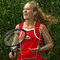Example single-person pose estimation algorithm applied to an image. Image Credit: “Microsoft Coco: Common Objects in Context Dataset”, https://cocodataset.org.

As stated before, the single-pose estimation algorithm is the simpler and faster of the two. Its ideal use case is for when there is only one person centered in an input image or video. The disadvantage is that if there are multiple persons in an image, keypoints from both persons will likely be estimated as being part of the same single pose — meaning, for example, that person #1’s left arm and person #2’s right knee might be conflated by the algorithm as belonging to the same pose. If there is any likelihood that the input images will contain multiple persons, the multi-pose estimation algorithm should be used instead.

Let’s review the inputs for the single-pose estimation algorithm:

• Input image element — An html element that contains an image to predict poses for, such as a video or image tag. Importantly, the image or video element fed in should be square.
• Image scale factor — A number between 0.2 and 1. Defaults to 0.50. What to scale the image by before feeding it through the network. Set this number lower to scale down the image and increase the speed when feeding through the network at the cost of accuracy.
• Flip horizontal — Defaults to false. If the poses should be flipped/mirrored horizontally. This should be set to true for videos where the video is by default flipped horizontally (i.e. a webcam), and you want the poses to be returned in the proper orientation.
• Output stride — Must be 32, 16, or 8. Defaults to 16. Internally, this parameter affects the height and width of the layers in the neural network. At a high level, it affects the accuracy and speed of the pose estimation. The lower the value of the output stride the higher the accuracy but slower the speed, the higher the value the faster the speed but lower the accuracy. The best way to see the effect of the output stride on output quality is to play with the single-pose estimation demo.

Now let’s review the outputs for the single-pose estimation algorithm:

• A pose, containing both a pose confidence score and an array of 17 keypoints.
• Each keypoint contains a keypoint position and a keypoint confidence score. Again, all the keypoint positions have x and y coordinates in the input image space, and can be mapped directly onto the image.

This short code block shows how to use the single-pose estimation algorithm:

const imageScaleFactor = 0.50;
const flipHorizontal = false;
const outputStride = 16;
const imageElement = document.getElementById('cat');// load the posenet model
const net = await posenet.load();
const pose = await net.estimateSinglePose(imageElement, scaleFactor, flipHorizontal, outputStride);

An example output pose looks like the following:

{
"score": 0.32371445304906,
"keypoints": [
{ // nose
"position": {
"x": 301.42237830162,
"y": 177.69162777066
},
"score": 0.99799561500549
},
{ // left eye
"position": {
"x": 326.05302262306,
"y": 122.9596464932
},
"score": 0.99766051769257
},
{ // right eye
"position": {
"x": 258.72196650505,
"y": 127.51624706388
},
"score": 0.99926537275314
},
...
]
}

Part 2b: Multi-person Pose Estimation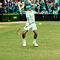Example multi-person pose estimation algorithm applied to an image. Image Credit: “Microsoft Coco: Common Objects in Context Dataset”, https://cocodataset.org

The multi-person pose estimation algorithm can estimate many poses/persons in an image. It is more complex and slightly slower than the single-pose algorithm, but it has the advantage that if multiple people appear in a picture, their detected keypoints are less likely to be associated with the wrong pose. For that reason, even if the use case is to detect a single person’s pose, this algorithm may be more desirable.

Moreover, an attractive property of this algorithm is that performance is not affected by the number of persons in the input image. Whether there are 15 persons to detect or 5, the computation time will be the same.

Let’s review the inputs:

• Input image element — Same as single-pose estimation
• Image scale factor — Same as single-pose estimation
• Flip horizontal — Same as single-pose estimation
• Output stride — Same as single-pose estimation
• Maximum pose detections — An integer. Defaults to 5. The maximum number of poses to detect.
• Pose confidence score threshold — 0.0 to 1.0. Defaults to 0.5. At a high level, this controls the minimum confidence score of poses that are returned.
• Non-maximum suppression (NMS) radius — A number in pixels. At a high level, this controls the minimum distance between poses that are returned. This value defaults to 20, which is probably fine for most cases. It should be increased/decreased as a way to filter out less accurate poses but only if tweaking the pose confidence score is not good enough.

The best way to see what effect these parameters have is to play with the multi-pose estimation demo.

Let’s review the outputs:

• A promise that resolves with an array of poses.
• Each pose contains the same information as described in the single-person estimation algorithm.

This short code block shows how to use the multi-pose estimation algorithm:

const imageScaleFactor = 0.50;
const flipHorizontal = false;
const outputStride = 16;
// get up to 5 poses
const maxPoseDetections = 5;
// minimum confidence of the root part of a pose
const scoreThreshold = 0.5;
// minimum distance in pixels between the root parts of poses
const nmsRadius = 20;
const imageElement = document.getElementById('cat');// load posenet
const net = await posenet.load();
const poses = await net.estimateMultiplePoses(
imageElement, imageScaleFactor, flipHorizontal, outputStride,

An example output array of poses looks like the following:

// array of poses/persons
[
{ // pose #1
"score": 0.42985695206067,
"keypoints": [
{ // nose
"position": {
"x": 126.09371757507,
"y": 97.861720561981
},
"score": 0.99710708856583
},
...
]
},
{ // pose #2
"score": 0.13461434583673,
"keypositions": [
{ // nose
"position": {
"x": 116.58444058895,
"y": 99.772533416748
},
"score": 0.9978438615799
},
...
]
},
...
]

If you’ve read this far, you know enough to get started with the PoseNet demos. This is probably a good stopping point. If you’re curious to know more about the technical details of the model and implementation, we invite you to continue reading below.

For Curious Minds: A Technical Deep Dive

In this section, we’ll go into a little more technical detail regarding the single-pose estimation algorithm. At a high level, the process looks like this: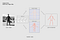Single person pose detector pipeline using PoseNet

One important detail to note is that the researchers trained both a ResNet and a MobileNet model of PoseNet. While the ResNet model has a higher accuracy, its large size and many layers would make the page load time and inference time less-than-ideal for any real-time applications. We went with the MobileNet model as it’s designed to run on mobile devices.

Revisiting the Single-pose Estimation Algorithm

Processing Model Inputs: an Explanation of Output Strides

First we’ll cover how to obtain the PoseNet model outputs (mainly heatmaps and offset vectors) by discussing output strides.

Conveniently, the PoseNet model is image size invariant, which means it can predict pose positions in the same scale as the original image regardless of whether the image is downscaled. This means PoseNet can be configured to have a higher accuracy at the expense of performance by setting the output stride we’ve referred to above at runtime.

The output stride determines how much we’re scaling down the output relative to the input image size. It affects the size of the layers and the model outputs. The higher the output stride, the smaller the resolution of layers in the network and the outputs, and correspondingly their accuracy. In this implementation, the output stride can have values of 8, 16, or 32. In other words, an output stride of 32 will result in the fastest performance but lowest accuracy, while 8 will result in the highest accuracy but slowest performance. We recommend starting with 16.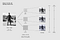The output stride determines how much we’re scaling down the output relative to the input image size. A higher output stride is faster but results in lower accuracy.

Underneath the hood, when the output stride is set to 8 or 16, the amount of input striding in the layers is reduced to create a larger output resolution. Atrous convolution is then used to enable the convolution filters in the subsequent layers to have a wider field of view (atrous convolution is not applied when the output stride is 32). While Tensorflow supported atrous convolution, TensorFlow.js did not, so we added a PR to include this.

Model Outputs: Heatmaps and Offset Vectors

When PoseNet processes an image, what is in fact returned is a heatmap along with offset vectors that can be decoded to find high confidence areas in the image that correspond to pose keypoints. We’ll go into what each of these mean in a minute, but for now the illustration below captures at a high-level how each of the pose keypoints is associated to one heatmap tensor and an offset vector tensor.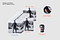Each of the 17 pose keypoints returned by PoseNet is associated to one heatmap tensor and one offset vector tensor used to determine the exact location of the keypoint.

Both of these outputs are 3D tensors with a height and width that we’ll refer to as the resolution. The resolution is determined by both the input image size and the output stride according to this formula:

Resolution = ((InputImageSize - 1) / OutputStride) + 1// Example: an input image with a width of 225 pixels and an output
// stride of 16 results in an output resolution of 15
// 15 = ((225 - 1) / 16) + 1

Heatmaps

Each heatmap is a 3D tensor of size resolution x resolution x 17, since 17 is the number of keypoints detected by PoseNet. For example, with an image size of 225 and output stride of 16, this would be 15x15x17. Each slice in the third dimension (of 17) corresponds to the heatmap for a specific keypoint. Each position in that heatmap has a confidence score, which is the probability that a part of that keypoint type exists in that position. It can be thought of as the original image being broken up into a 15x15 grid, where the heatmap scores provide a classification of how likely each keypoint exists in each grid square.

Offset Vectors

Each offset vector is a 3D tensor of size resolution x resolution x 34, where 34 is the number of keypoints * 2. With an image size of 225 and output stride of 16, this would be 15x15x34. Since heatmaps are an approximation of where the keypoints are, the offset vectors correspond in location to the heatmap points, and are used to predict the exact location of the keypoints as by traveling along the vector from the corresponding heatmap point. The first 17 slices of the offset vector contain the x of the vector and the last 17 the y. The offset vector sizes are in the same scale as the original image.

Estimating Poses from the Outputs of the Model

After the image is fed through the model, we perform a few calculations to estimate the pose from the outputs. The single-pose estimation algorithm for example returns a pose confidence score which itself contains an array of keypoints (indexed by part ID) each with a confidence score and x, y position.

To get the keypoints of the pose:

1. A sigmoid activation is done on the heatmap to get the scores.
scores = heatmap.sigmoid()
2. argmax2d is done on the keypoint confidence scores to get the x and y index in the heatmap with the highest score for each part, which is essentially where the part is most likely to exist. This produces a tensor of size 17x2, with each row being the y and x index in the heatmap with the highest score for each part.
heatmapPositions = scores.argmax(y, x)
3. The offset vector for each part is retrieved by getting the x and y from the offsets corresponding to the x and y index in the heatmap for that part. This produces a tensor of size 17x2, with each row being the offset vector for the corresponding keypoint. For example, for the part at index k, when the heatmap position is y and d, the offset vector is:
offsetVector = [offsets.get(y, x, k), offsets.get(y, x, 17 + k)]
4. To get the keypoint, each part’s heatmap x and y are multiplied by the output stride then added to their corresponding offset vector, which is in the same scale as the original image.
keypointPositions = heatmapPositions * outputStride + offsetVectors
5. Finally, each keypoint confidence score is the confidence score of its heatmap position. The pose confidence score is the mean of the scores of the keypoints.

Multi-person Pose Estimation

The details of the multi-pose estimation algorithm are outside of the scope of this post. Mainly, that algorithm differs in that it uses a greedy process to group keypoints into poses by following displacement vectors along a part-based graph. Specifically, it uses the fast greedy decoding algorithm from the research paper PersonLab: Person Pose Estimation and Instance Segmentation with a Bottom-Up, Part-Based, Geometric Embedding Model. For more information on the multi-pose algorithm please read the full research paper or look at the code.

It’s our hope that as more models are ported to TensorFlow.js, the world of machine learning becomes more accessible, welcoming, and fun to new coders and makers. PoseNet on TensorFlow.js is a small attempt at making that possible. We’d love to see what you make — and don’t forget to share your awesome projects using #tensorflowjs and #posenet!

Written by

TensorFlow

TensorFlow is an end-to-end open source platform for machine learning.

Welcome to a place where words matter. On Medium, smart voices and original ideas take center stage - with no ads in sight. Watch
Follow all the topics you care about, and we’ll deliver the best stories for you to your homepage and inbox. Explore
Get unlimited access to the best stories on Medium — and support writers while you’re at it. Just \$5/month. Upgrade